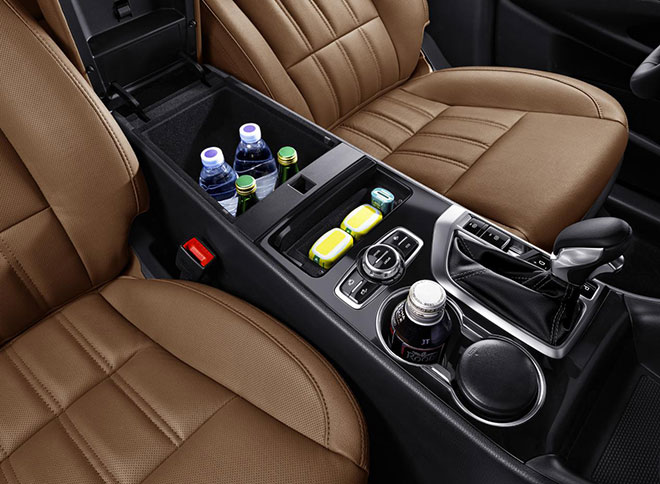## 二胎家庭 这辆7座的大SUV能满足你所有需求

2017年08月16日 11:07 来源：车行天下 超过：15226次关注

二胎政策全面放开以来，越来越多的人选择生二孩。多个孩子，家里多份热闹，这对家庭来说是好事。但我们都知道，一个政策的出台影响不止一面，而是多个方面的，比如对汽车市场来说，就催生了人们购车时对7座车的需求，而空间大、通过性强的7座SUV，也自然成为越来越多的二孩家庭买车的首要选择。如何选择一辆合适的7座SUV，自然成为二孩家庭面前的难题，市场上关于车的信息那么多，有的还是水军发布的，究竟哪一辆车才是真的好？对于消费者来说也是丈二的和尚，摸不着头脑。今天，小编就为大家良心推荐一款好用又实在的车，满足二孩家庭的各方面需求，它就是来自长安的CS95。家里多了新成员，自然对车的空间要求更大！7座是首选，但市场上的7座SUV的空间大小也各有差距。长安CS95拥有4949*1930*1785mm的阔绰车身及2810mm的超长轴距，其阔适多变的座椅功能组合，能全面满足全路况全场景出行所需，让你从此避免家庭出行人多不够坐的尴尬。同时，基于人机工程的全局设计，更让每一排都有充足纵向头部空间、腿部空间和横向肩肘臀空间，为乘员营造出舒适的驾乘体验。此外，车内还贴心设计了38处储物空间，加上二三排座椅放平后可达2米深的后备空间，即使小孩东西再多，CS95也能最大限度满足二孩家庭出行时对行李储放的需求。有了二孩后带上全家出游，可谓是人生中最幸福的时刻，工作中的烦恼都顺势一扫而空。而这时如果车的动力不给力，幸福感就要大打则扣了。动力方面，CS95搭载了一台历时六年，由长安自主研发的蓝鲸2.0TGDI发动机，其最大功率为171KW(233Ps), 最大扭矩为360N·m，百公里加速仅需8.41S；与之匹配的爱信新一代6AT变速器最大可承载450N·m的扭矩，0.8s内即可完成换挡响应；还有博格华纳全新一代NexTrac 智能四驱电子控制系统，匹配现代岱莫斯免维护PTU和后桥；黄金动力三剑客的搭配，令CS95拥有优秀的脱困能力，即使家庭出游面对复杂路况，操控也能畅快如一。小孩子，天性爱玩，当然需要更丰富的智能互联服务才能释放其天性，满足他们的好奇心，也是让行车更便捷的一大法宝。CS95搭载in Call智能车载互联系统，拥有车载Wi-Fi热点，可分享给随行乘客，让小孩能够玩自己喜欢的游戏，实现一路上网畅游。

此外，开车的家长，可在车内收听新闻、听音乐等打发行车无聊的闲暇时光。更贴心的是，CS95具备强大的语音控制能力，你只需动一动嘴巴，即可控制导航、天窗、空调、多媒体、电话，还可以语音搜索在线天气、股票、新闻等生活资讯，是不是非常方便！此外，CS95还拥有自适应巡航、自动泊车、车道偏离预警、360度全景影像、PAB预警辅助制动系统、全景天窗和日本先锋牌音箱等丰富配置，再加上15.98万-22.98万的售价，可谓是物超所值，对于有二孩的或者准备生二孩的家庭来说，都是一个非常不错的选择。

#### 相关文章

0-500 字已有评论 0条 查看评论>>

### 热门标签

﻿
• 快速找车
• 选择品牌
• 选择品牌
• A  奥迪
• A  阿斯顿·马丁
• A  阿尔法·罗密欧
• B  宝沃
• B  布加迪
• B  巴博斯
• B  保时捷
• B  宾利
• B  奔驰
• B  宝马
• B  本田
• B  别克
• B  标致
• B  比亚迪
• B  宝骏
• B  北汽制造
• B  北汽新能源
• B  北汽幻速
• B  北汽威旺
• B  北京汽车
• B  奔腾
• B  北汽绅宝
• C  长安
• C  长安商用
• C  长城
• C  昌河
• D  大众
• D  道奇
• D  DS
• D  东南
• D  东风风神
• D  东风风行
• D  东风小康
• D  东风风度
• D  东风
• F  福特
• F  丰田
• F  菲亚特
• F  法拉利
• F  福田
• F  福迪
• F  福汽启腾
• G  观致
• G  广汽传祺
• G  广汽吉奥
• G  GMC
• H  红旗
• H  汉腾汽车
• H  哈弗
• H  哈飞
• H  海格
• H  海马
• H  华颂
• H  黄海
• H  华泰
• H  恒天
• J  吉利汽车
• J  捷豹
• J  Jeep
• J  江淮
• J  江铃
• J  金杯
• J  九龙
• J  金旅
• K  凯翼
• K  凯迪拉克
• K  克莱斯勒
• K  科尼塞克
• K  卡威
• K  开瑞
• L  路虎
• L  林肯
• L  劳斯莱斯
• L  兰博基尼
• L  雷克萨斯
• L  铃木
• L  雷诺
• L  理念
• L  力帆
• L  莲花汽车
• L  猎豹
• L  路特斯
• L  陆风
• M  马自达
• M  MG
• M  MINI
• M  玛莎拉蒂
• M  摩根
• M  迈凯轮
• N  纳智捷
• O  欧宝
• O  讴歌
• O  欧朗
• Q  奇瑞
• Q  起亚
• Q  启辰
• R  日产
• R  荣威
• R  瑞麒
• S  三菱
• S  斯威汽车
• S  萨博
• S  smart
• S  斯柯达
• S  斯巴鲁
• S  思铭
• S  双龙
• S  上汽大通
• S  双环
• T  特斯拉
• T  腾势
• W  沃尔沃
• W  五菱汽车
• W  五十铃
• W  威兹曼
• W  威麟
• X  现代
• X  雪佛兰
• X  雪铁龙
• X  西雅特
• Y  一汽
• Y  英菲尼迪
• Y  英致
• Y  依维柯
• Y  野马汽车
• Y  永源
• Z  众泰
• Z  中华
• Z  中兴
• Z  知豆
• 选择车系
• 选择车系
• 车型对比
• 选择品牌
• 选择品牌
• A  奥迪
• A  阿斯顿·马丁
• A  阿尔法·罗密欧
• B  宝沃
• B  布加迪
• B  巴博斯
• B  保时捷
• B  宾利
• B  奔驰
• B  宝马
• B  本田
• B  别克
• B  标致
• B  比亚迪
• B  宝骏
• B  北汽制造
• B  北汽新能源
• B  北汽幻速
• B  北汽威旺
• B  北京汽车
• B  奔腾
• B  北汽绅宝
• C  长安
• C  长安商用
• C  长城
• C  昌河
• D  大众
• D  道奇
• D  DS
• D  东南
• D  东风风神
• D  东风风行
• D  东风小康
• D  东风风度
• D  东风
• F  福特
• F  丰田
• F  菲亚特
• F  法拉利
• F  福田
• F  福迪
• F  福汽启腾
• G  观致
• G  广汽传祺
• G  广汽吉奥
• G  GMC
• H  红旗
• H  汉腾汽车
• H  哈弗
• H  哈飞
• H  海格
• H  海马
• H  华颂
• H  黄海
• H  华泰
• H  恒天
• J  吉利汽车
• J  捷豹
• J  Jeep
• J  江淮
• J  江铃
• J  金杯
• J  九龙
• J  金旅
• K  凯翼
• K  凯迪拉克
• K  克莱斯勒
• K  科尼塞克
• K  卡威
• K  开瑞
• L  路虎
• L  林肯
• L  劳斯莱斯
• L  兰博基尼
• L  雷克萨斯
• L  铃木
• L  雷诺
• L  理念
• L  力帆
• L  莲花汽车
• L  猎豹
• L  路特斯
• L  陆风
• M  马自达
• M  MG
• M  MINI
• M  玛莎拉蒂
• M  摩根
• M  迈凯轮
• N  纳智捷
• O  欧宝
• O  讴歌
• O  欧朗
• Q  奇瑞
• Q  起亚
• Q  启辰
• R  日产
• R  荣威
• R  瑞麒
• S  三菱
• S  斯威汽车
• S  萨博
• S  smart
• S  斯柯达
• S  斯巴鲁
• S  思铭
• S  双龙
• S  上汽大通
• S  双环
• T  特斯拉
• T  腾势
• W  沃尔沃
• W  五菱汽车
• W  五十铃
• W  威兹曼
• W  威麟
• X  现代
• X  雪佛兰
• X  雪铁龙
• X  西雅特
• Y  一汽
• Y  英菲尼迪
• Y  英致
• Y  依维柯
• Y  野马汽车
• Y  永源
• Z  众泰
• Z  中华
• Z  中兴
• Z  知豆
• 选择车系
• 选择车系
• 选择车型
• 选择车型
• 意见反馈